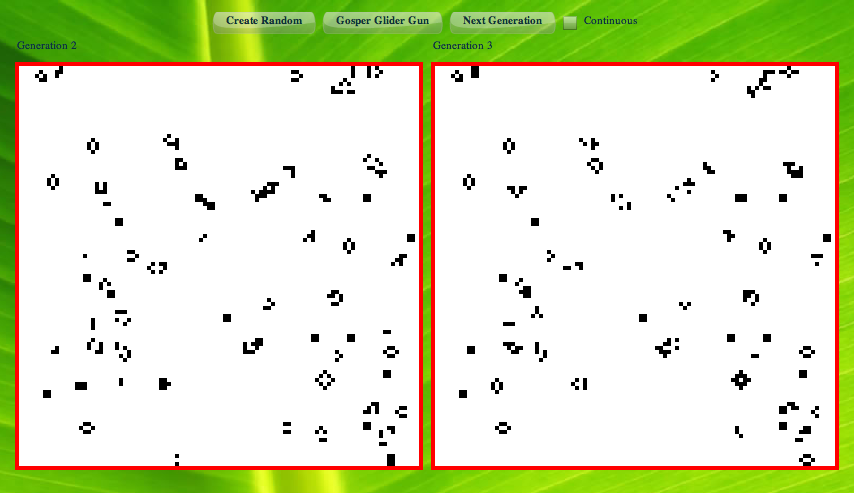### Pixel Bender and Conway's Game of Life

When I started reading about Pixel Bender and realized at its core it was a cellular automaton engine I immediately thought of Conway's Game of Life. I started looking into how to use Pixel Bender to render generations in the game of life.

Given that pixel bender is boundless (i.e. defined over an infinite plane of discrete pixel coordinates) I had to introduce the notion of a border pixel to make handling edge cases easier. Since most of the area in a generation is dead I decided to use white for a pixel that is dead (easier on the eyes) and black for a pixel that was alive. My initial attempt at the algorithm ran into a bug with the kernel export for Flash Player feature. After some rewriting I had a working pixel bender kernel that implemented the logic (albeit unoptimized):

```<languageVersion : 1.0;>
// \$Id: GameOfLife.pbk 57 2008-09-16 02:55:33Z danielr \$
kernel GameOfLife
<   namespace : "Daniel Rinehart";
vendor : "NeoPhi.com";
version : 1;
description : "Conway's Game of Life";
>
{
input image4 src;
output pixel4 dst;

void
evaluatePixel()
{
pixel4 border = pixel4(1.0, 0.0, 0.0, 1.0);
pixel4 dead = pixel4(1.0, 1.0, 1.0, 1.0);
pixel4 alive = pixel4(0.0, 0.0, 0.0, 1.0);

pixel4 me = sampleNearest(src, outCoord());

// default to no change in pixel
dst = me;

// I would like to write "all(equal(border, me))" but that's buggy in Flash
if (!((border.r == me.r) && (border.g == me.g) && (border.b == me.b)))
{
int aliveNeighborCount = 0;
float2 offset = float2(pixelSize(src).x, pixelSize(src).y);

// Find out how many of my neighbors are alive
// left
pixel4 test = sampleNearest(src, outCoord() + (offset * float2(-1.0, 0.0)));
if ((alive.r == test.r) && (alive.g == test.g) && (alive.b == test.b))
{
aliveNeighborCount++;
}
// upper left
test = sampleNearest(src, outCoord() + (offset * float2(-1.0, -1.0)));
if ((alive.r == test.r) && (alive.g == test.g) && (alive.b == test.b))
{
aliveNeighborCount++;
}
// up
test = sampleNearest(src, outCoord() + (offset * float2(0.0, -1.0)));
if ((alive.r == test.r) && (alive.g == test.g) && (alive.b == test.b))
{
aliveNeighborCount++;
}
// upper right
test = sampleNearest(src, outCoord() + (offset * float2(1.0, -1.0)));
if ((alive.r == test.r) && (alive.g == test.g) && (alive.b == test.b))
{
aliveNeighborCount++;
}
// right
test = sampleNearest(src, outCoord() + (offset * float2(1.0, 0.0)));
if ((alive.r == test.r) && (alive.g == test.g) && (alive.b == test.b))
{
aliveNeighborCount++;
}
// lower right
test = sampleNearest(src, outCoord() + (offset * float2(1.0, 1.0)));
if ((alive.r == test.r) && (alive.g == test.g) && (alive.b == test.b))
{
aliveNeighborCount++;
}
// down
test = sampleNearest(src, outCoord() + (offset * float2(0.0, 1.0)));
if ((alive.r == test.r) && (alive.g == test.g) && (alive.b == test.b))
{
aliveNeighborCount++;
}
// lower left
test = sampleNearest(src, outCoord() + (offset * float2(-1.0, 1.0)));
if ((alive.r == test.r) && (alive.g == test.g) && (alive.b == test.b))
{
aliveNeighborCount++;
}

// As per: http://en.wikipedia.org/wiki/Conway%27s_Game_of_Life
// 1. Any live cell with fewer than two live neighbours dies, as if by loneliness.
// 2. Any live cell with more than three live neighbours dies, as if by overcrowding.
// 3. Any live cell with two or three live neighbours lives, unchanged, to the next generation.
if (((alive.r == me.r) && (alive.g == me.g) && (alive.b == me.b)) && ((aliveNeighborCount < 2) || (aliveNeighborCount > 3)))
{
}
// 4. Any dead cell with exactly three live neighbours comes to life.
{
dst = alive;
}
}
}
}```

Now with a working filter I used some of the recent posts by Mike Chambers on how to embed a pixel bender kernel into MXML. However, since I wasn't using it as a filter and wanted more control over the flow I leveraged the ShaderJob class.

```var shader:Shader = new Shader(new gameOfLife() as ByteArray);
var result:BitmapData = new BitmapData(current.width, current.height);